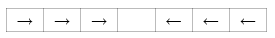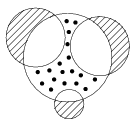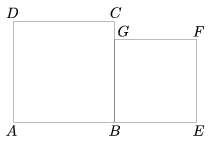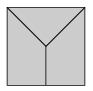Mathematical and Physical Journal
for High Schools
Issued by the MATFUND Foundation
 Already signed up? New to KöMaL?

# KöMaL Problems in Mathematics, September 2007

Show/hide problems of signs:## Problems with sign 'K'

Deadline expired on October 10, 2007.

K. 127. Every digit of a four-digit positive integer is multiplied by four, and a four- digit number is obtained that is four times the original number. How many such four-digit numbers are there?

(6 pont)

solution (in Hungarian), statistics

K. 128. The starting position of a logical game is shown below:The pieces marked with arrows are moving. Every piece may move in the direction indicated by the arrow on it: it may either move to the adjoining vacant field or skip to the next field over any piece in the adjoining field, provided that the next field is free. The task is to make the counters marked with the two kinds of arrows exchange places. Draw the sequence of moves to achieve the exchange.

(6 pont)

solution (in Hungarian), statistics

K. 129. At the beginning of the new schoolyear Pete started to print the sequence

200720082007200820072008...

on his computer. The printer however ran out of ink after the 2007th digit has been printed. The amount of ink to print a digit 2 is 2/3 of the amount to print a 0 or 8, while printing a digit 7 requires half the amount of ink to print a 2. How many 7's could have been printed using the original amount of ink in the printed?

(6 pont)

solution (in Hungarian), statistics

K. 130. There are 4 circles on the figure. Two of them have radius 2 and the smallest one has radius 1. What is the radius of the largest circle, if the area of the dashed part is equal to the area of the dotted part?(6 pont)

solution (in Hungarian), statistics

K. 131. There is a rectangular food box on the kitchen counter. Its outer dimensions are 3 dm×5 dm×2.9 dm. The box is standing on its 3×5-dm face. An ant is crawling from one lower corner to the diagonally opposite top corner on the outer surface of the box. (It cannot get in the interior of the box.) What is the shortest distance that it needs to cover?

(6 pont)

solution (in Hungarian), statistics

K. 132. How many six-digit numbers are there in which the sum of the squares of the digits is 9?

(6 pont)

solution (in Hungarian), statistics## Problems with sign 'C'

Deadline expired on October 15, 2007.

C. 905. Ann writes down two arbitrary natural numbers that consist of the same digits in different orders. She subtracts the smaller number from the greater one, and multiplies the difference by an arbitrary natural number. Then she deletes a non-zero digit from the product. She tells the remaining number to Bill. With a little thinking, Bill can guess the deleted digit. How?

(5 pont)

solution (in Hungarian), statistics

C. 906. The sides of a right-angled triangle form an arithmetic progression. Determine the ratios of the sides. Prove that the radius of the inscribed circle is the common difference of the arithmetic progression.

(5 pont)

solution (in Hungarian), statistics

C. 907. The square ABCD of side a and the square BEFG of side b are drawn next to each other as shown in the figure. Express in terms of a and b the area of the quadrilateral formed by the midpoints of the line segments AB, BE, FC and DG.(5 pont)

solution (in Hungarian), statistics

C. 908. A group of ten people went to the cinema. They bought tickets to 5 consecutive seats in each of two different rows. Al and Ben wanted to sit next to each other, while Sue and Ann did not want to sit next to each other. How many different seatings were possible?

(5 pont)

solution (in Hungarian), statistics

C. 909. The lengths of the sides of a triangle are whole numbers. One of them is 7, and the opposite angle is 60o. What may be the area of the triangle?

(Suggested by B. Kovács, Szatmárnémeti)

(5 pont)

solution (in Hungarian), statistics## Problems with sign 'B'

Deadline expired on October 15, 2007.

B. 4012. Alice has 8 keys on a ring. The keys look all alike, and both sides of them are the same. In order to be able to distinguish them, Alice puts a coloured cap on each key. How many colours does she need?

(3 pont)

solution (in Hungarian), statistics

B. 4013. Is it possible to colour the positive rational numbers in red and blue so that

(a) the sum of red numbers is red and the sum of blue numbers is blue;

(b) the product of red numbers is red and the product of blue numbers is blue?

(4 pont)

solution (in Hungarian), statistics

B. 4014. A', B' and C', respectively, are the reflections of the vertices of a triangle ABC about the centre of the circumscribed circle. Prove that the sum of the areas of the triangles A'BC, AB'C, and ABC' equals the area of the triangle ABC.

(3 pont)

solution (in Hungarian), statistics

B. 4015. The diagonals of a convex quadrilateral enclose an angle of 45o. Drop a perpendicular from each vertex of the quadrilateral onto the line of the diagonal connecting the two adjacent vertices. Find the ratio of the area of the quadrilateral formed by the feet of the perpendiculars to the area of the original quadrilateral.

(Suggested by J. Bodnár, Budapest)

(3 pont)

solution (in Hungarian), statistics

B. 4016. In every group of 9 out of a company of 12 people, there are 5 people who pairwise know each other. Prove that there are 6 members of the company who pairwise know each other, too.

(5 pont)

solution (in Hungarian), statistics

B. 4017. The circle k2 touches the circle k1 on the inside at point A. The tangent drawn to circle k2 at a point D different from A intersects the circle k1 at the points B and C. Prove that AD bisects the angle BAC.

(4 pont)

solution (in Hungarian), statistics

B. 4018. P is a given point on the tangent drawn at point B of a circle of diameter AB. The point of tangency of the other tangent from P is C. T is the orthogonal projection of C on the line AB. Prove that AP bisects the line segment CT.

(4 pont)

solution (in Hungarian), statistics

B. 4019. Prove thatfor every positive integer n.

(Competition problem from the Highlands)

(4 pont)

solution (in Hungarian), statistics

B. 4020. Find two non-congruent polyhedra that both have the front elevation and plan shown below in the figure. (The common point in the inside is the centre of the square. The line segments of the squares are all visible edges, but there are no other invisible edges either.)(5 pont)

solution (in Hungarian), statistics

B. 4021. Prove that if each of the numbers a1,a2,...,an is at least 1, then

(a1+1)(a2+1).....(an+1)2n-1(a1+a2+...+an-n+2).

(4 pont)

solution (in Hungarian), statistics## Problems with sign 'A'

Deadline expired on October 15, 2007.

A. 431. Triangle ABC is not isosceles. The incenter is I, the excenter is O. The incircle touches the sides BC, CA, AB at points D, E, F, respectively. Lines FD and AC intersect at P, lines DE and AB intersect at Q. The midpoints of segments EP amd FQ are M and N, respectively. Prove that MN and OI are perpendicular.

(5 pont)

solution, statistics

A. 432. Find all integer a for which there exists distinct positive integers x and y such that (axy+1) divides (ax2+1)2.

(5 pont)

solution, statistics

A. 433. Prove that if a, b, c are real numbers such that a2+b2+c2=1 then.

(Proposed by János Bodnár, Budapest)

(5 pont)

solution, statistics